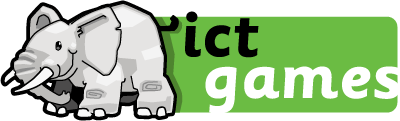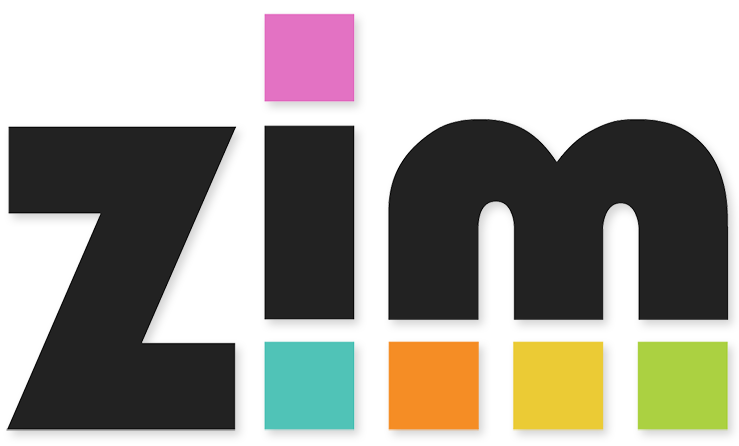# Abacus

### Demonstrator for Counting, Place Value & Decimals.

Learning Objectives:
• Y1: To count to and across 100, forwards and backwards, beginning with 0 or 1, or from any given number
• Y2: To recognise the place value of each digit in a two-digit number (tens, ones)
• Y3: To recognise the place value of each digit in a three-digit number (hundreds, tens, ones)
• Y3: To read and write numbers up to 1000 in numerals [and in words]
• Y4: To find 1000 more or less than a given number
• Y4: To recognise the place value of each digit in a four-digit number (thousands, hundreds, tens, and ones)
• Y5: To read, write, [order and compare numbers] to at least 1 000 000 and determine the value of each digit
• Y5: To count forwards or backwards in steps of powers of 10 for any given number up to 1 000 000
• Y5: To recognise and use thousandths and relate them to tenths, hundredths and decimal equivalents
• Y5: To read, write, [order and compare] numbers with up to three decimal places
• Y6: To read, write, [order and compare] numbers up to 10 000 000 and determine the value of each digit
• Y6: To identify the value of each digit in numbers given to three decimal places and multiply and divide numbers by 10, 100 and 1000 giving answers up to three decimal places
A simple interactive abacus. Great fo modelling counting forwards/backwards & place value.

### Instructions:

Press the [ + ] button to count forwards in steps of 1,10,100 etc.

Press the [ - ] button to count backwards in steps of 1,10,100 etc.

Why not press [ num ] and hide the digits. Then ask the children to write the number on their drywipe boards?

Close your eyes and listen as we add tens.... write the number we have reached.

Press [ dec ] to see decimal numbers. The triangles move the decimal place.

Have fun!

Build on the learning with
these games:Made using theandlibraries.